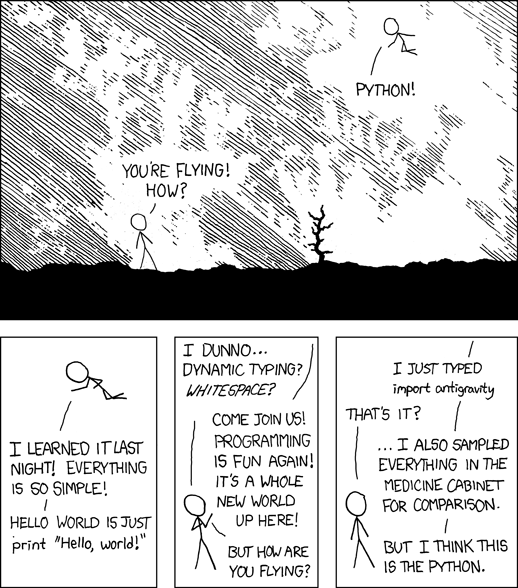# Mahotas and the Python Ecosystem for Bioimage Informatics Applications

Luis Pedro Coelho (EMBL) — Main developer of mahotas

## Python has a growing ecosystem of scientific packages around numpy

Numpy provides basic data types (arrays, matrices).
Packages provide intelligence.

## The wider ecosystem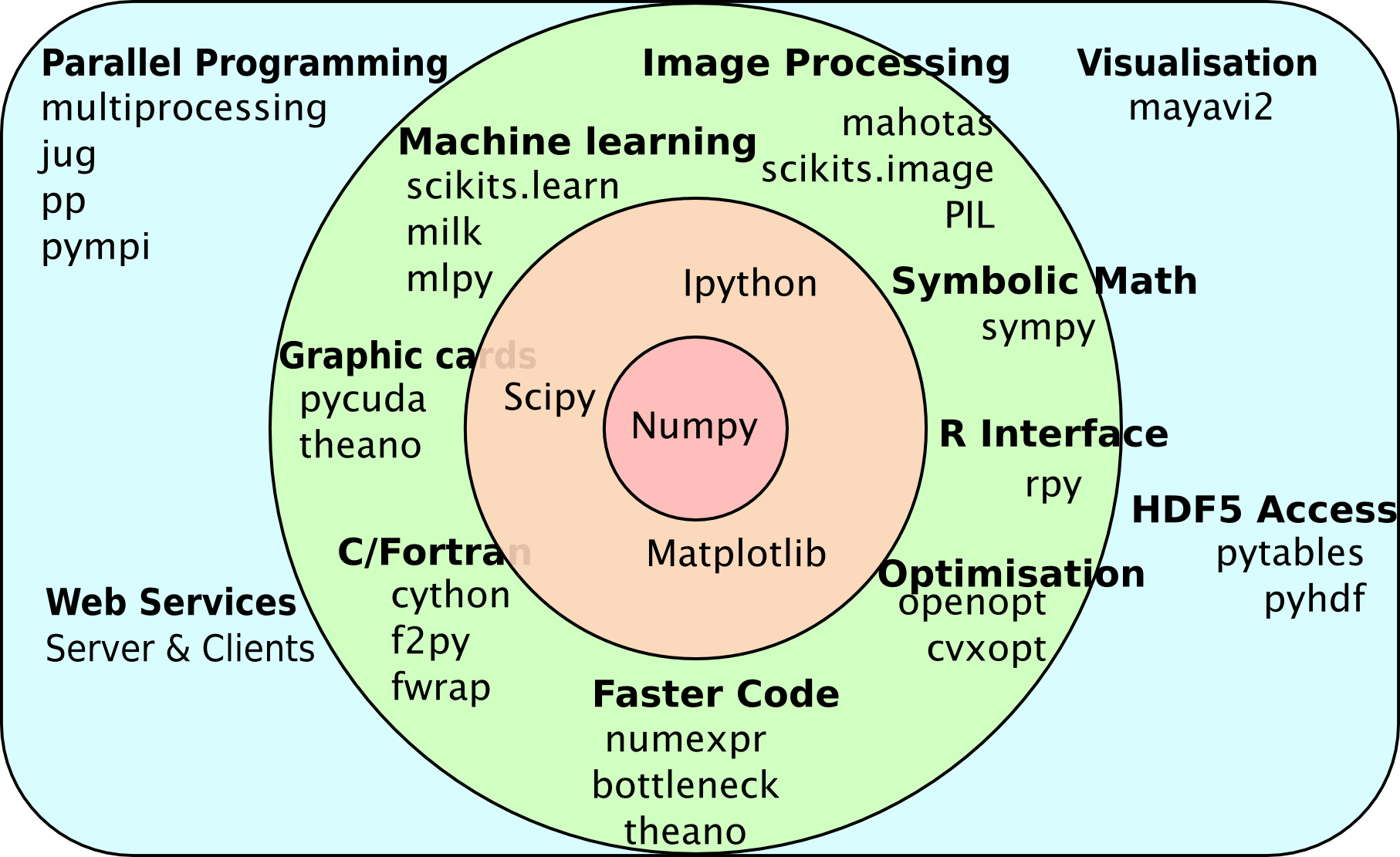## Multiple packages act together

### Mahotas can rely on pre-existing functionality

1. An image type (numpy array).
2. Types to hold computed data (numpy array again).
3. Plotting & displaying (matplotlib).
4. Machine learning (sklearn or milk).

## Modularity is good software engineering

• Improvements to one package benefit all.
• Separation of concerns.

## Consistency also helps human users

• Single type for many uses.
• Many simple operations can be done in numpy.
• Same basic conventions.
• No copying/conversion of data between packages.

## What can mahotas do for you?

(including formats like LSM or STK).
• Image filtering (morphological, Gaussian, &c)
• Feature computation (Haralick, LBPs, SURF, &c)
• Most functions work in 3D (or even 4D, 5D, up to 32D).
• Many utility functions (total is over 100 functions)

## Mahotas' internal code is in C++

• It is also heavily optimized
• The same is true of other scientific packages
(Or they use C or Fortran)

## Mahotas is general purpose, but was developed for bioimage informatics

Original goal:
to support the Murphy Lab pipeline with Python.

Has since grown.

## A worked out example of using mahotas to classify some images

2. Compute features
3. Use machine learning

I'm going to use a
subcellular determination problem as an example.

## Example of cytoplasmic class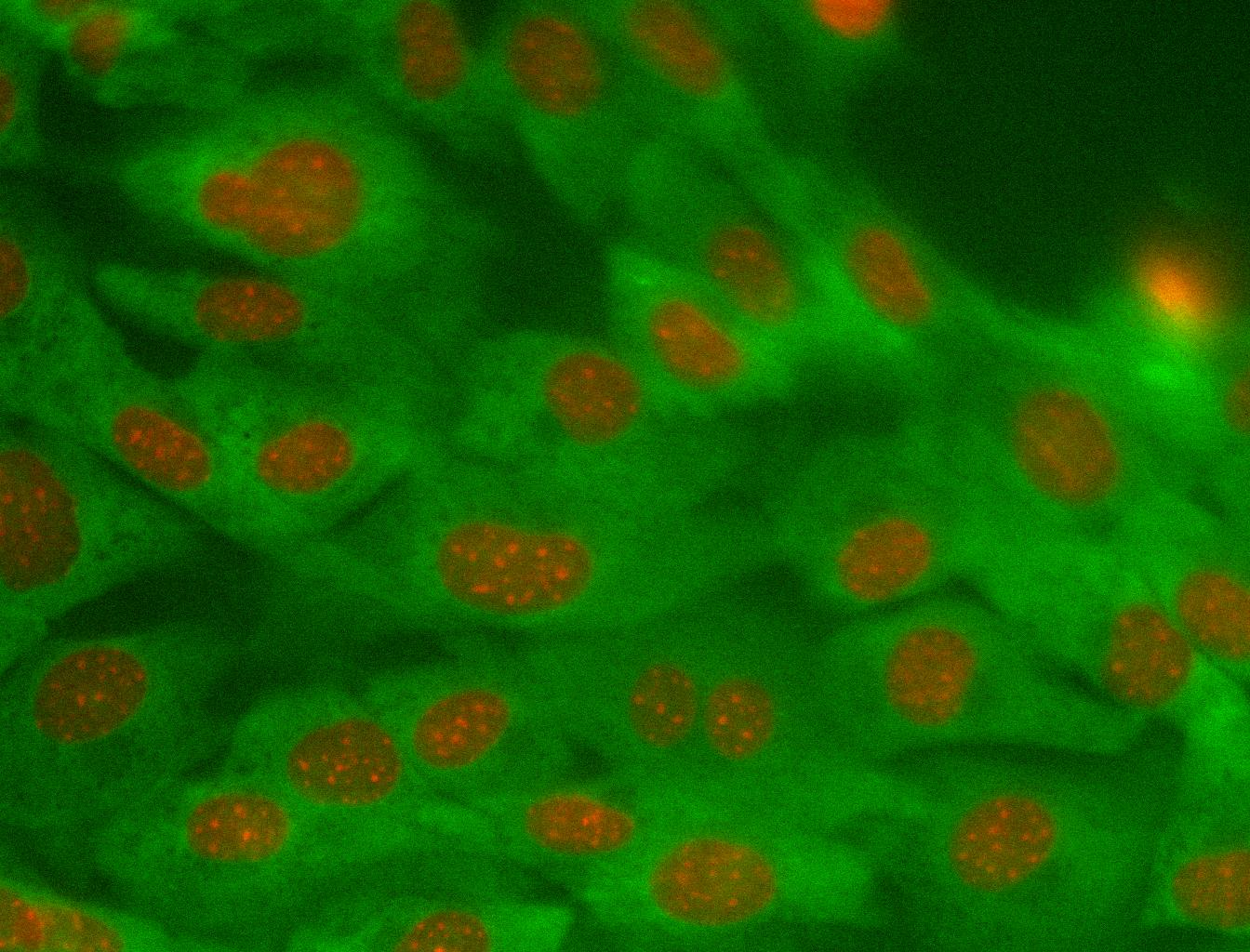## Example of nuclear class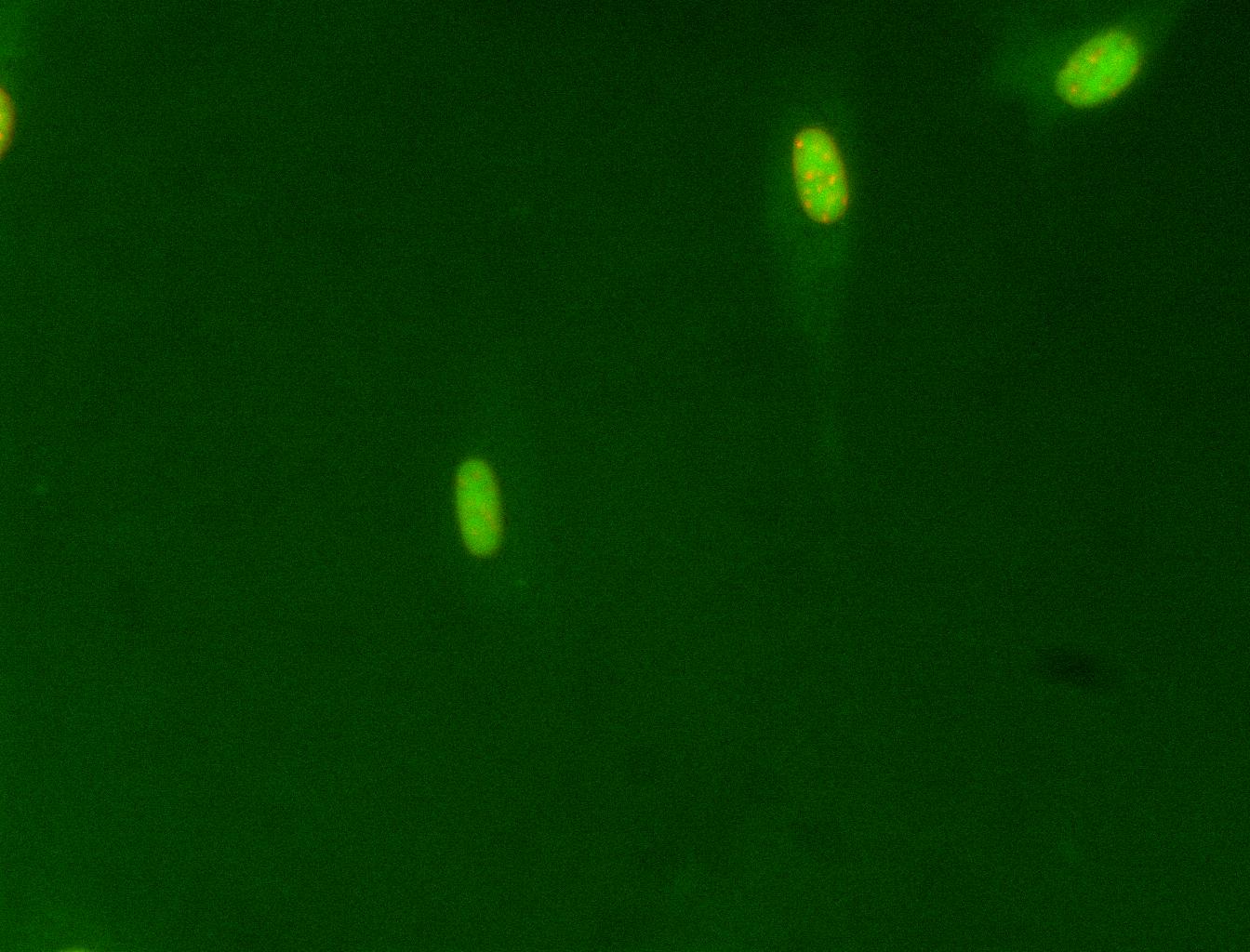## Example of code (I): imports & init

``````
from glob import glob
import numpy as np
import mahotas as mh
``````
``````

images = glob('nuclear/*dna.tiff') + glob('cytoplasmic/*dna.tiff')
labels = []
features = []
``````

## Example of code (II): compute features

``````
for im in images:
features.append(mh.features.haralick(protein).mean(0))
labels.append('nuclear' in im)

features = np.array(features)
labels = np.array(labels)
``````

## Example of code (III): call sklearn

``````

from sklearn import cross_validation
from sklearn.linear_model.logistic import LogisticRegression
scores = cross_validation.cross_val_score(
LogisticRegression(), features, labels, cv=5)
print("Logistic regression accuracy: {:%}".format(scores.mean()))
``````

## Example of code (IV): call milk

``````
import milk
cmat,_ = milk.nfoldcrossvalidation(features, labels)

acc = cmat.trace()/float(cmat.sum())

print("SVM accuracy: {:%}".format(acc))
``````

## Let's See A Live Demonstration...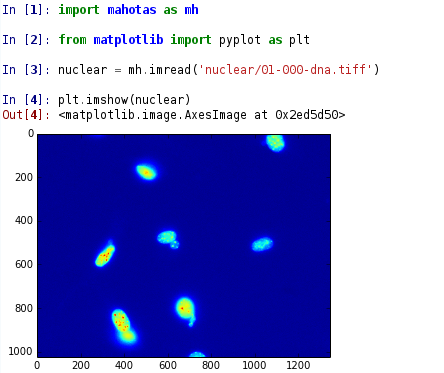## If you're coming from Matlab, Spyder will look familiar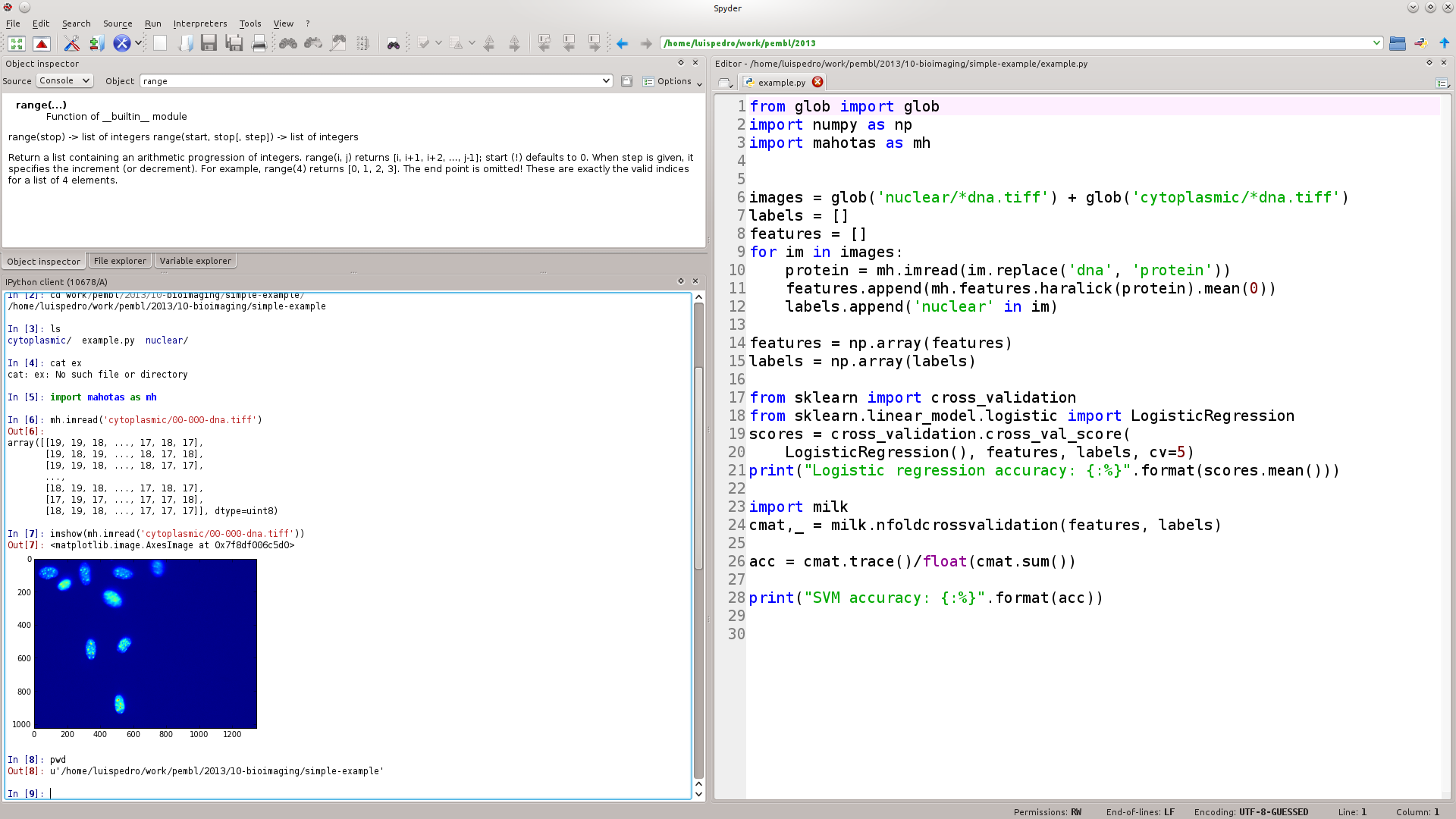## Basic packages are stable, others are expanding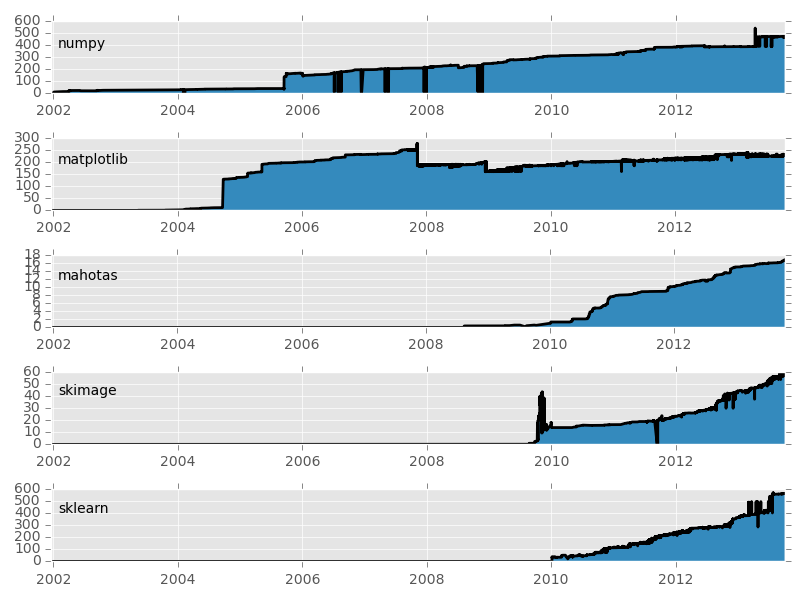## Mahotas releases...

• Version 1.0.3 October 6th
• Version 1.0.2. July 10th
• Version 1.0.1. July 9th
• Version 1.0. May 21st
• Version 0.99. April 22nd
• Version 0.9.7. February 3rd
• Version 0.9.6. December 2nd
• ...

## People are using it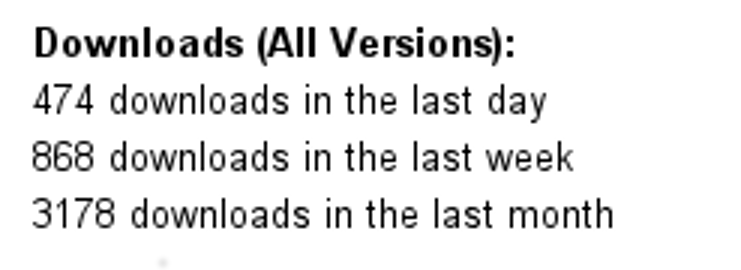## Mahotas has unit tests with automated testing

• Unit tests check for code quality and regression.
• Continuous integration (with travis).
• There are no known bugs in a release.

## Summary

• The Python Numpy-based ecosystem is powerful and flexible.
• Mahotas is the cog that was built for bioimage informatics.
• There is a lot of development activity.
• All the packages mentioned are open source (MIT-style).
• Commercial support is available.